# Construction of Bézier CurvesGiven n+1 points P0, P1, P2, ... and Pn in space, the control points, the Bézier curve defined by these control points iswhere the coefficients are defined as follows:Therefore, the point that corresponds to u on the Bézier curve is the "weighted" average of all control points, where the weights are the coefficients Bn,i(u). The line segments P0P1, P1P2, ..., Pn-1Pn, called legs, joining in this order form a control polyline. Many authors prefer to call this control polyline as control polygon. Functions Bn,i(u), 0 <= i <= n, are referred to as the Bézier basis functions or Bernstein polynomials.

Note that the domain of u is [0,1]. As a result, all basis functions are non-negative. In the above, since u and i can both be zero and so do 1 - u and n - i, we adopt the convention that 00 is 1. The following shows a Bézier curve defined by 11 control points, where the blue dot is a point on the curve that corresponds to u=0.4. As you can see in the figure, the curve more or less follows the polyline.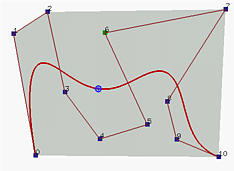The following properties of a Bézier curve are important:

1. The degree of a Bézier curve defined by n+1 control points is n:
In each basis function, the exponent of u is i + (n - i) = n. Therefore, the degree of the curve is n.
2. C(u) passes through P0 and Pn:
This is shown in the above figure. The curve passes though the first and the last control point. Please verify this yourself with some simple algebraic manipulation.
3. Non-negativity:
All basis functions are non-negative. We have mentioned this earlier.
4. Partition of Unity:
The sum of the basis functions at a fixed u is 1. It is not difficult to verify that the basis functions are the coefficients in the binomial expansion of the expression 1 = (u + (1 - u))n. Hence, their sum is one. Moreover, since they are nonnegative, we conclude that the value of any basis function is in the range of 0 and 1.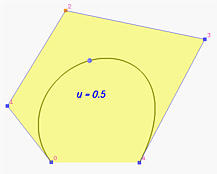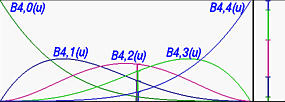In the left figure above, we have a Bézier curve defined by five control points. Its five basis functions are functions in u as shown in the right figure above. The figures show u=0.5 and all five basis functions. The right-most vertical bar shows the way of partitioning 1 into five intervals and hence the name of partition of unity. Note that the color of a partition is identical to the color used to draw its corresponding basis functions.

Since all basis functions are in the range of 0 an 1 and sum to one, they can be considered as weights in the computation of a weighted average. More precisely, we could say "to compute C(u), one takes the weight Bn,i(u) for control point Pi and sum them together."

5. Convex Hull Property:
This means the Bézier curve defined by the given n + 1 control points lies completely in the convex hull of the given control points. The convex hull of a set of points is the smallest convex set that contains all points. In the following figure, the convex hull of the 11 control points is shown in color gray. Note that not all control points are on the boundary of the convex hull. For example, control points 3, 4, 5, 6, 8 and 9 are in the interior. The curve, except for the first two endpoints, lies completely in the convex hull.This property is important because we are guaranteed that the generated curve will be in an understood and computable region and will not go outside of it.

6. Variation Diminishing Property:
If the curve is in a plane, this means no straight line intersects a Bézier curve more times than it intersects the curve's control polyline.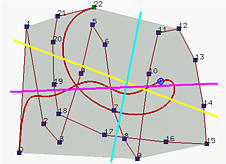Take a look at the above figure. The yellow line intersects the curve 3 times and the polyline 7 times; the magenta line intersects the curve 5 times and the polyline 7 times; and the cyan line intersects the curve and its polyline twice. You could draw other straight lines to verify this property.

If the curve is a space curve, replacing "line" with "plane" will suffice. Special cases may occur; however, it will not be a difficult task to come up with a proper counting scheme that takes these special cases into consideration.

So, what is the meaning of this property? It tells us that the complexity (i.e., turning and twisting) of the curve is no more complex than the control polyline. In other words, the control polyline twists and turns more frequently than the Bézier curve does, because an arbitrary line hits the control polyline more often than it hits the curve. Take a look at the above figure,the control polyline is more complex than the curve it defines.

7. Affine Invariance:
If an affine transformation is applied to a Bézier curve, the result can be constructed from the affine images of its control points. This is a nice property. When we want to apply a geometric or even affine transformation to a Bézier curve, this property states that we can apply the transformation to control points, which is quite easy, and once the transformed control points are obtained the transformed Bézier curve is the one defined by these new points. Therefore, we do not have to transform the curve.### What If the Domain of u Is Not [0.1]?

Sometimes the domain of a Bézier curve is [a,b] rather than [0,1]. Thus, a change of variable is required. What we should do is simply converting a u in [a,b] to a new u in [0,1] and using this u in the basis functions. Converting u to [0,1] can be done as follows: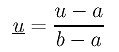Plugging this u into the basis function Bn,i(u) gives the following: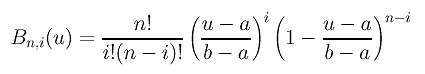These new basis functions define a Bézier curve on the domain of [a,b]. We shall use this fact in later sections.### A Short Summary

In summary, to define a Bézier curve of degree n, we need to choose n + 1 control points in space so that they roughly indicate the shape of the desired curve. Then, if it is not up to our expectation, we can move the control points around. As one or more control points are moved, the shape of the Bézier curve changes accordingly. But, the curve always lies in the convex hull defined by the control points (the convex hull property), and the shape of generated curve is less complex than the control polyline (variation diminishing property).